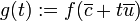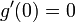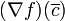Point of local extremum implies critical point for a function of multiple variables

This article describes a test that can be used to determine whether a point in the domain of a function gives a point of local, endpoint, or absolute (global) maximum or minimum of the function, and/or to narrow down the possibilities for points where such maxima or minima occur.
View a complete list of such tests
This article describes an analogue for functions of multiple variables of the following term/fact/notion for functions of one variable: point of local extremum implies critical point

Statement

Suppose$f$ is a function of a vector variable$\overline{x}$. Suppose$\overline{c}$ is a point in the interior of the domain of$f$, i.e.,$f$ is defined in an open ball containing$\overline{c}$.

Suppose further that$\overline{c}$ is a point of local extremum for$f$, i.e.,$f$ attains a local extreme value (maximum or minimum) at$\overline{c}$.

Then the following are true:

• For every linear direction at$\overline{c}$, the directional derivative of$f$ in that direction at$\overline{c}$ is either zero or it doesn't exist.
• Thus, the gradient vector of$f$ at$\overline{c}$ is either zero or it doesn't exist, i.e.,$f$ has a critical point at$\overline{c}$.

Facts used

1. Point of local extremum implies critical point (the single variable case)
2. Relation between gradient vector and directional derivatives

Proof

Directional derivative version

Given: A function$f$ of a vector variable$\overline{x}$. A point$\overline{c}$ in the interior of the domain where it attains a local extreme value. A unit vector$\overline{u}$.

To prove: The directional derivative$\nabla_{\overline{u}}(f)(\overline{c})$ equals zero or it doesn't exist.

Proof:

Step no. Assertion/construction Facts used Given data used Previous steps used Explanation
1 Consider the following function of one variable:$g(t) := f(\overline{c} + t\overline{u})$. In other words,$g$ is the restriction of$f$ to a line through$\overline{c}$ along the direction of$\overline{u}$.
2$g$ has a local extreme value at$t = 0$, which corresponds to the value$f(\overline{c})$. Step (1) Since$f$ has a local extreme value at$\overline{c}$,$g$ (a function of one variable) has a local extreme value of the same type at$t = 0$.
3$g$ has a critical point at 0, i.e.,$g'(0) = 0$ or$g'(0)$ doesn't exist. Fact (1) Step (2) Fact-step combination direct
4 The directional derivative$\nabla_{\overline{u}}(f)(\overline{c})$ equals zero or it doesn't exist. Definition of directional derivative Step (3) One of the definitions of directional derivative is as the ordinary derivative$g'(0)$. Thus, this follows directly from Step (3).

Given: A function$f$ of a vector variable$\overline{x}$. A point$\overline{c}$ in the interior of the domain where it attains a local extreme value.
To prove: The gradient vector$(\nabla f)(\overline{c})$ is either zero or it doesn't exist.
1 For every unit vector$\overline{u}$, the directional derivative$\nabla_{\overline{u}}(f)(\overline{c})$ equals zero or it doesn't exist. Directional derivative version of proof
2 If the gradient vector$(\nabla f)(\overline{c})$ exists, then for every unit vector$\overline{u}$, the directional derivative$\nabla_{\overline{u}}(f)(\overline{c})$ exists and equals$\overline{u} \cdot (\nabla f)(\overline{c})$. Fact (2)
3 If the gradient vector$(\nabla f)(\overline{c})$ exists, then for every unit vector$\overline{u}$, we have$\overline{u} \cdot (\nabla f)(\overline{c}) = 0$ Steps (1), (2) Step-combination direct
4 If the gradient vector$(\nabla f)(\overline{c})$ exists, it must equal the zero vector. Any vector whose dot product with every vector is zero must be the zero vector. Step (3) Step-fact combination direct